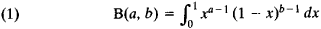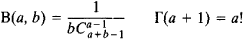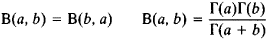# Euler's Integral

The following article is from The Great Soviet Encyclopedia (1979). It might be outdated or ideologically biased.

## Euler’s Integral

The integralis known as Euler’s integral of the first kind or the beta function. It was studied by L. Euler in 1730 and 1731 and had been previously considered by I. Newton and J. Wallis.

The integralis known as Euler’s integral of the second kind or the gamma function. It was considered by Euler in 1729 and 1730 in a form equivalent to equation (2); equation (2) itself was given by Euler in 1781.

The name “Euler’s integral” was introduced by A. Legendre. Such integrals make it possible to generalize to the case of continuously varying arguments the binomial coefficientsand the factorial n!: if a and b are natural numbers, thenIntegrals (1) and (2) converge absolutely if a and b are positive; they do not exist if a and b are negative. The following relations hold:The second relation expresses the beta function in terms of the gamma function. There are a number of relations between Euler’s integrals, for different values of the argument, that generalize the corresponding relations between the binomial coefficients. Euler’s integrals also may be considered for complex values of the arguments a and b. Euler’s integrals are encountered in many problems of the theory of special functions; many definite integrals that do not have an elementary expression reduce to Euler’s integrals.

The name “Euler’s integral” is also sometimes applied to the integralwhich expresses the hypergeometric function.

### REFERENCES

Fikhtengol’ts, G. M. Kurs differentsial’nogo i integral’nogo ischisleniia, 7th ed., vol. 2. Moscow, 1969.
Artin, E. Vvedenie v teoriiu gamma-funktsii. Moscow-Leningrad, 1934. (Translated from German.)
Whittaker, E. T., and G. N. Watson. Kurs sovremennogo analiza, 2nd ed., part 2. Moscow, 1963. (Translated from English.)